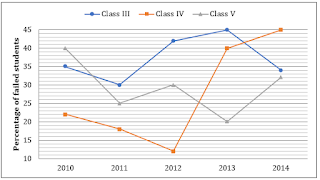# LIC AAO Quantitative Aptitude Miscellaneous Quiz: 10th April 2019

Dear Aspirants,

Quantitative Aptitude Quiz For LIC AAO
Numerical Ability or Quantitative Aptitude Section has given heebie-jeebies to the aspirants when they appear for a banking examination. As the level of every other section is only getting complex and convoluted, there is no doubt that this section, too, makes your blood run cold. The questions asked in this section are calculative and very time-consuming. But once dealt with proper strategy, speed, and accuracy, this section can get you the maximum marks in the examination. Following is the Quantitative Aptitude quiz to help you practice with the best of latest pattern questions.

Directions (1-5): Find out the approximate value which should replace the question mark (?) in the following questions. (You are not expected to find out the exact value)

Q1. 447.75 ÷ 28 × 4.99 = ?

60
70
72
80
75

Q2. 1679 ÷ 14.95 × 5.02 = ?

540
525
545
560
520

Q3. 5237.897 – 6629.010 + 7153.999 – 2205.102 = ?

6340
4688
5240
3558
6290

Q4. 459% of 849.947 + 266.5% of 6284.012 – 1486.002 = ?

20330
12640
15000
22160
19140

Q5. (9321 + 5406 + 1001) ÷ (498 + 929 + 660) = ?

6
4
18
8
12

Q6. Quantity I: Percentage mark-up above cost price of an article so as to gain 33% after allowing the customer a discount of 5%.
Quantity II: Percentage of dancers under 25 years out of a group of 20 singers and 40 dancer if 20% of the singers are under 25 years old and 40% of the entire group is under 25 years.

Quantity I > Quantity II
Quantity I < Quantity II
Quantity I ≥ Quantity II
Quantity I ≤ Quantity II
Quantity I = Quantity II or No relation

Q7. Quantity I: Value of fifth number when Average of five numbers is 61. The average of 1st and 3rd number is 69 and average of second and fourth number is 69.
Quantity II: No. of boys in the class. The average age of all students of a class is 18 years. The average age of boys of the class is 20 years and that of the girls is 15 years. The no. of girls in the class is 20.
Note: Compare the magnitudes of quantities.

Quantity I > Quantity II
Quantity I < Quantity II
Quantity I ≥ Quantity II
Quantity I ≤ Quantity II
Quantity I = Quantity II or No relation

Q8. A 20 litres mixture contains milk and water in the respective ratio of 3 : 2. Then 10 litres of the mixture is removed and replaced with pure milk and the operation is repeated once more. At the end of the two removals and replacements, what is the ratio of milk and water in the resultant mixture respectively ?

17 : 3
9 : 1
4 : 17
5 : 3
3 : 14

Q9. On walking at 3/4 of his usual speed a man reaches his office 20 minutes late. What is the usual time taken by him in reaching his office ?

75 minutes
60 minutes
40 minutes
30 minutes
None of these

Q10. A wholesaler blends two varieties of tea, one costing Rs 60 per kilo and another costing Rs 105 per kilo. The respective ratio of quantities they were mixed in was 7 : 2. If he sold the mixed variety of Rs 100 per kilo, how much was his profit percentage?

Directions (11-15): The following graph shows the percentage of failed students in three different classes of a school in five different years.
Study the graph carefully and answer the following questions.Q11. In 2012, in class V, 140 students passed which is equal to 175% of failed students of same class in 2013. Find the ratio of failed students and passed students in 2012 & 2013 respectively in class V.

5 : 16
3 : 16
4 : 17
3 : 17
None of these

Q12. If the total strength of students in 2010 in class IV was 150 and it increases every year by 50, then find the number of passed students in 2013 in class IV.

182
160
180
170
155

Q13. The ratio of girls to boys who failed in class III in 2014 was 7 : 10. One-seventh i.e. 5 of these girls passed when their answer sheet were re-evaluated which made the number of passed girls i.e. 84 in 2014, 20% more than those in 2010 in same class. Find the number of passed boys in 2010 in class III. Total students in class III in 2014 and 2010 are in ratio 5 : 4.

60
65
70
62
None of these

Q14. In 2012, 105 students failed in class III, while 198 students passed in class IV. The number of passed boys is 17 more than the passed girls in class III and the number of failed girls is 15 less than the failed boys in class IV. Find the difference in failed boys of class IV and passed girls of class III.

45
50
52
43
None of these

Q15.Find the average of percentage of passed students of class IV throughout the given years

76.2%
70.8%
72.6%
70.2%
None of these

You May also like to Read: